## Extra Questions of Class 10 Maths Chapter 6 Triangles PDF Download

We have provided you with Extra and Important Questions from Class 10 Maths Chapter 6 Triangles. This Extra and Important Questions will help you to score 100% in your Board Exams. These extra questions will be helpful to revise the important topics and concepts.

# Triangles Class 10 Important Questions with Answers Maths Chapter 6

## Extra Questions for Class 10 Maths Chapter 6 Very Short Answer Type

Question:Two sides and the perimeter of one triangle are respectively three times the corresponding sides and the perimeter of the other triangle. Are the two triangles similar? Why?

Answer:Since the perimeters and two sides are proportional
∴ The third side is proportional to the corresponding third side.
i.e., The two triangles will be similar by SSS criterion.

Question:A and B are respectively the points on the sides PQ and PR of a ∆PQR such that PQ = 12.5 cm, PA = 5 cm, BR = 6 cm, and PB = 4 cm. Is AB || QR? Give reason.

Question:∆DEF ~ ∆ABC, if DE : AB = 2 : 3 and ar(∆DEF) is equal to 44 square units. Find the area (∆ABC).

Question:Is the triangle with sides 12 cm, 16 cm and 18 cm a right triangle? Give reason.

Answer:Here, 122+ 162 = 144 + 256 = 400 ≠ 182
∴ The given triangle is not a right triangle.

Question:In triangles PQR and TSM, ∠P = 55°, ∠Q = 25°, ∠M = 100°, and ∠S = 25°. Is ∆QPR ~ ∆TSM? Why?

Answer:Şince, ∠R = 180° – (∠P + ∠Q)
= 180° – (55° + 25°) = 100° = ∠M
∠Q = ∠S = 25° (Given)
∆QPR ~ ∆STM
i.e., . ∆QPR is not similar to ∆TSM.

Question:If ABC and DEF are similar triangles such that ∠A = 47° and ∠E = 63°, then the measures of ∠C = 70°. Is it true? Give reason.

∴ ∠A = ∠D = 47°
∠B = ∠E = 63°
∴ ∠C = 180° – (∠A + ∠B) = 180° – (47° + 63°) = 70°
∴ Given statement is true.

Question:Let ∆ABC ~ ∆DEF and their areas be respectively 64 cm2 and 121 cm2. If EF = 15.4 cm, find BC.

Question:ABC is an isosceles triangle right-angled at C. Prove that AB2 = 2AC2.

∴ AB2 = AC2 + BC2 [By Pythagoras theorem]
⇒ AB2 = AC2 + AC2
[∵ AC = BC]
⇒ AB2 = 2AC2

## Extra Questions for Class 10 Maths Chapter 6 Short Answer Type

Question:Sides of triangle are given below. Determine which of them are right triangles. In case of a right triangle, write the length of its hypotenuse.
(i) 7cm, 24 cm, 25 cm
(ii) 3 cm, 8 cm, 6 cm

(i) Let a = 7 cm, b = 24 cm and c = 25 cm.
Here, largest side, c = 25 cm
We have, a2 + b2 = (7)2 + (24)2 = 49 + 576 = 625 = c2 [∵c = 25]
So, the triangle is a right triangle.
Hence, c is the hypotenuse of right triangle.

(ii) Let a = 3 cm, b = 8 cm and c = 6 cm
Here, largest side, b = 8 cm
We have, a2 + c2 = (3)2 + (6)2 = 9 + 36 = 45 ≠ b2
So, the triangle is not a right triangle.

Question:If triangle ABC is similar to triangle DEF such that 2AB = DE and BC = 8 cm. Then find the length of EF.

∆ABC ~ ∆DEF (Given)

Question:In an isosceles ∆ABC, if AC = BC and AB2 = 2AC2, then find ∠C.

Answer: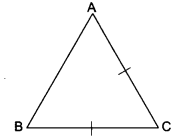AB2 = 2AC2 (Given)
AB2 = AC2 + AC2
AB2 = AC2 + BC2 (∵ AC = BC)
Hence AB is the hypotenuse and ∆ABC is a right angle A.
So, ∠C = 90°

Question:

The length of the diagonals of a rhombus are 16 cm and 12 cm. Find the length of side of the rhombus.

Answer:∵ The diagonals of rhombus bisect each other at 90°.
∴ In the right angle ∆BOC
BO = 8 cm
CO = 6 cm
∴ By Pythagoras Theorem
BC2 = BO2 + CO2 = 64 + 36
BC2 = 100
BC = 10 cm

Question:A man goes 24 m towards West and then 10 m towards North. How far is he from the starting point?

By Pythagoras Theorem
AC2 = AB2 + BC2 = (24)2 + (10)2
AC2 = 676
AC = 26 m
∴ The man is 26 m away from the starting point.

Question:∆ABC ~ ∆DEF such that AB = 9.1 cm and DE = 6.5 cm. If the perimeter of ∆DEF is 25 cm, what is the perimeter of ∆ABC?

Since ∆ABC ~ ∆DEF.

Question:∆ABC ~ ∆PQR; if area of ∆ABC = 81 cm2, area of ∆PQR = 169 cm2 and AC = 7.2 cm, find the length of PR.

Since ∆ABC ~ ∆PQR

Question:E and F are points on the sides PQ and PR respectively of a ∆PQR. Show that EF ||QR if PQ = 1.28 cm, PR= 2.56 cm, PE = 0.18 cm and PF = 0.36 cm.

Solution: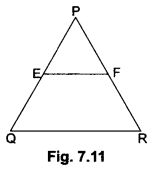We have, PQ = 1.28 cm, PR = 2.56 cm
PE = 0.18 cm, PF = 0.36 cm
Now, EQ = PQ-PE = 1.28 – 0.18 = 1.10 cm and
FR = PR – PF = 2.56 – 0.36 = 2.20 cm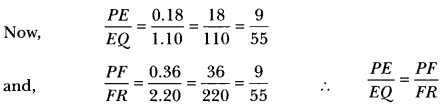Therefore, EF || QR [By the converse of Basic Proportionality Theorem]

Question:A vertical pole of length 6 m casts a shadow 4 m long on the ground and at the same time a tower casts a shadow 28 m long. Find the height of the tower.

Let AB be a vertical pole of length 6m and BC be its shadow and DE be tower and EF be its shadow. Join AC and DF.
Now, in ∆ABC and ∆DEF, we have

h = 42 Hence, height of tower, DE = 42m

Question:

State which pairs of triangles in the following figures are similar. Write the similarity criterion used by you for answering the question and also write the pairs of similar triangles in the symbolic form.

(i) In ∆ABC and ∆QRP, we have

∆NML is not similar to ∆PQR.

## Extra Questions for Class 10 Maths Chapter 6 Long Answer Type

Question:In Fig. 7.37, ABCD is a trapezium with AB || DC. If ∆AED is similar to ΔBEC, prove that AD = BC.

In ∆EDC and ∆EBA we have
∠1 = ∠2 [Alternate angles]
∠3 = ∠4 [Alternate angles]
∠CED = ∠AEB [Vertically opposite angles]
∴ ∆EDC ~ ∆EBA [By AA criterion of similarity]

Question:Prove that the area of an equilateral triangle described on a side of a right-angled isosceles triangle is half the area of the equilateral triangle described on its hypotenuse.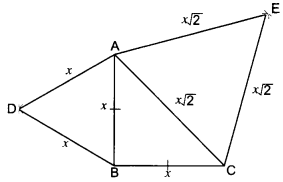Given: A ∆ABC in which ∠ABC = 90° and AB = BC.
∆ABD and ΔCAE are equilateral triangles.
To Prove: ar(∆ABD) = 1/2 × ar(∆CAE)
Proof: Let AB = BC = x units.
∴ hyp. CA = √x2 + √x2 = x√2 units.
Each of the ABD and ∆CAE being equilateral has each angle equal to 60°.
∴ ∆АВD ~ ∆CAE
But, the ratio of the areas of two similar triangles is equal to the ratio of the squares of their corresponding sides.

Question:If the areas of two similar triangles are equal, prove that they are congruent.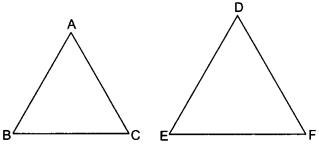Given: Two triangles ABC and DEF, such that
∆ABC ~ ∆DEF and area (∆ABC) = area (∆DEF)
To prove: ∆ABC ≅ ∆DEF
Proof: ∆ABC ~ ∆DEF
⇒ ∠A = ∠D, ∠B = ∠E, ∠C = ∠F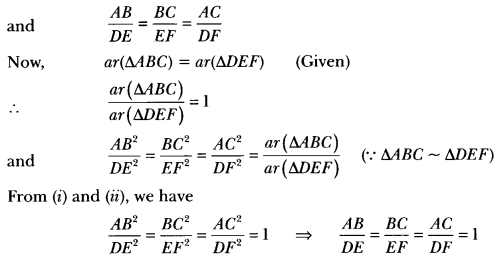AB = DE, BC = EF, AC = DF
∆ABC ≅ ∆DEF (By SSS criterion of congruency)

Question:Prove that the ratio of the areas of two similar triangles is equal to the square of the ratio of their corresponding medians.

Let ∆ABC and ∆PQR be two similar triangles. AD and PM are the medians of ∆ABC and ∆PQR respectively.

Question:The perpendicular from A on side BC of a ∆ABC intersects BC at D such that DB = 3CD (see Fig. 7.42). Prove that 2AB2 = 2AC2 + BC2We have, DB = 3CD
Now,
BC = BD + CD
⇒ BC = 3CD + CD = 4CD (Given DB = 3CD)
∴ CD = 14 BC
and DB = 3CD = 14BC
Now, in right-angled triangle ABD using Pythagoras Theorem we have
AB2 = AD2 + DB2 …(i)
Again, in right-angled triangle ∆ADC, we have
AC2 = AD2 + CD2 …(ii)
Subtracting (ii) from (i), we have
AB2 – AC2 = DB2 – CD2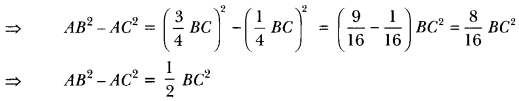∴ 2AB2 – 2AC2 = BC2
⇒ 2AB2 = 2AC2 + BC2